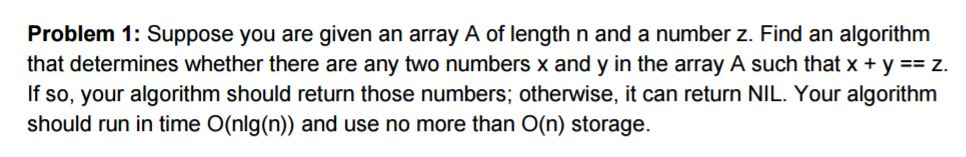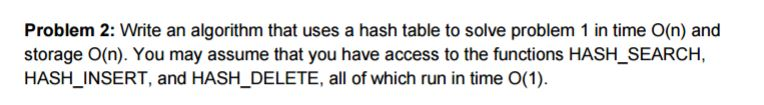# Question & Answer: Suppose you are given an array A of length n and a number z. Find an algorithm that determ…..#include <stdio.h>

Don't use plagiarized sources. Get Your Custom Essay on
Question & Answer: Suppose you are given an array A of length n and a number z. Find an algorithm that determ…..
GET AN ESSAY WRITTEN FOR YOU FROM AS LOW AS \$13/PAGE

#include <iostream>

#include <unordered_set>

using namespace std;

void findPairs(int arr[], int size, int sum)

{

int flag = 1;

unordered_set<int> us;

for(int i=0; i<size; i++)

{

int diff = sum-arr[i];

if(us.find(arr[i])==us.end())

us.insert(diff);

else

{

printf(“Two numbers in the array with given sum %d is (%d, %d)n”, sum, arr[i], diff);

flag = 0;

}

}

if(flag == 1)

printf(“No such sum found.n”);

}

int main()

{

int arr[] = {10, 20, 38, 17, 7, 11};

int n = 24;

int size = sizeof(arr)/sizeof(arr);

findPairs(arr, size, n);

return 0;

}Suppose you are given an array A of length n and a number z. Find an algorithm that determines whether there are any two numbers x and y in the array A such that x + y == z. If so, your algorithm should return those numbers: otherwise, it can return NIL. Your algorithm should run in time O(nlg(n)) and use no more than O(n) storage. Write an algorithm that uses a hash table to solve problem 1 in time O(n) and storage O(n). You may assume that you have access to the functions HASH_SEARCH, HASH_INSERT, and HASH_DELETE, all of which run in time O(1).

// solution for problem 1 is already priovided.

// problem 2 solution using hashmap

// C code using hashmap to find sum pair in O(n) time
#include <stdio.h>
#include <stdbool.h>
#define MAX 100000

void findPairs(int arr[], int size, int sum)
{
int t;
bool hashMap[MAX] = {0};

for (int i = 0; i < size; i++)
{
t = sum – arr[i];
if (t >= 0 && hashMap[t] == 1)
printf(“Pair with given sum %d is (%d, %d) n”, sum, arr[i], t);
hashMap[arr[i]] = 1;
}
}

int main()
{
int arr[] = {10, 20, 38, 17, 7, 11};
int n = 24;
int size = sizeof(arr)/sizeof(arr);
findPairs(arr, size, n);
return 0;
}